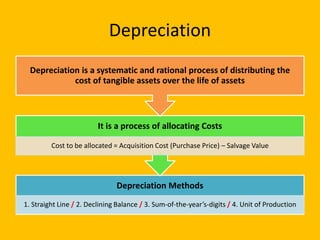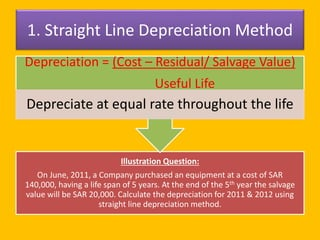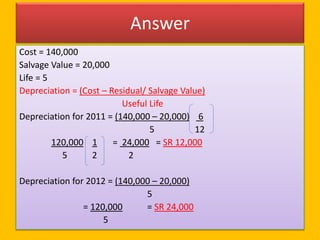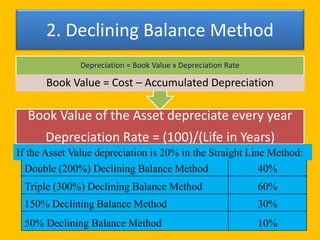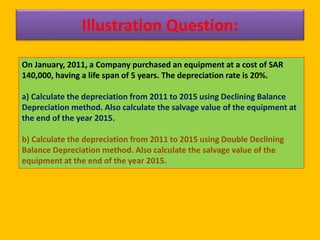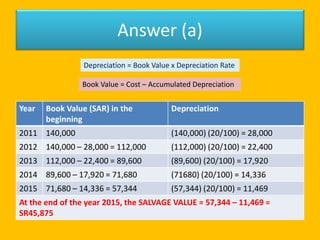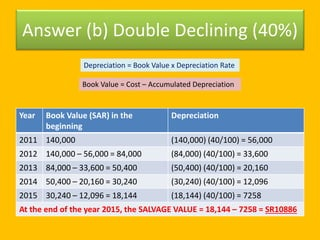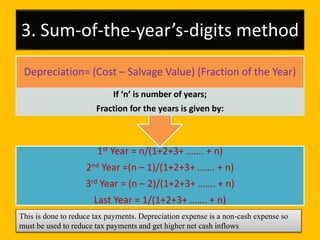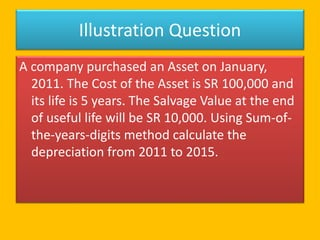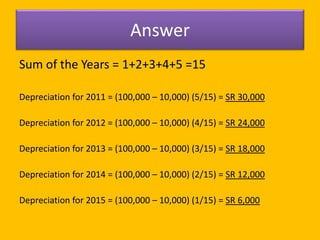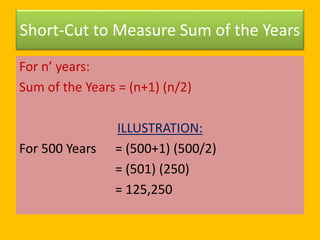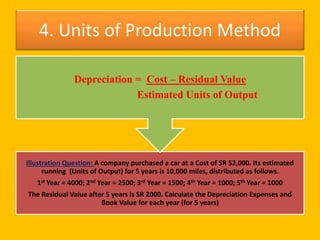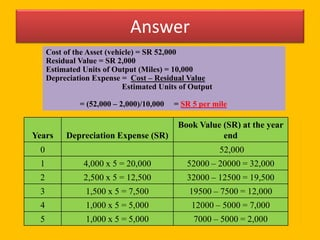1 de 13Methods of depreciationAashish Singh8 depreciationextrakillerDepreciation accounting completeRam samAccounting rate of returnKristel Pamela CaluyaDepreciation complete concept and methods sarmadalikamboh

### Depreciation methods

1. Depreciation Depreciation Methods 1. Straight Line / 2. Declining Balance / 3. Sum-of-the-year’s-digits / 4. Unit of Production It is a process of allocating Costs Cost to be allocated = Acquisition Cost (Purchase Price) – Salvage Value Depreciation is a systematic and rational process of distributing the cost of tangible assets over the life of assets
2. 1. Straight Line Depreciation Method Illustration Question: On June, 2011, a Company purchased an equipment at a cost of SAR 140,000, having a life span of 5 years. At the end of the 5th year the salvage value will be SAR 20,000. Calculate the depreciation for 2011 & 2012 using straight line depreciation method. Depreciation = (Cost – Residual/ Salvage Value) Useful Life Depreciate at equal rate throughout the life
3. Answer Cost = 140,000 Salvage Value = 20,000 Life = 5 Depreciation = (Cost – Residual/ Salvage Value) Useful Life Depreciation for 2011 = (140,000 – 20,000) 6 5 12 120,000 1 = 24,000 = SR 12,000 5 2 2 Depreciation for 2012 = (140,000 – 20,000) 5 = 120,000 = SR 24,000 5
4. 2. Declining Balance Method Book Value of the Asset depreciate every year Depreciation Rate = (100)/(Life in Years) Depreciation = Book Value x Depreciation Rate Book Value = Cost – Accumulated Depreciation Double (200%) Declining Balance Method 40% Triple (300%) Declining Balance Method 60% 150% Declining Balance Method 30% 50% Declining Balance Method 10% If the Asset Value depreciation is 20% in the Straight Line Method:
5. Illustration Question: On January, 2011, a Company purchased an equipment at a cost of SAR 140,000, having a life span of 5 years. The depreciation rate is 20%. a) Calculate the depreciation from 2011 to 2015 using Declining Balance Depreciation method. Also calculate the salvage value of the equipment at the end of the year 2015. b) Calculate the depreciation from 2011 to 2015 using Double Declining Balance Depreciation method. Also calculate the salvage value of the equipment at the end of the year 2015.
6. Answer (a) Year Book Value (SAR) in the beginning Depreciation 2011 140,000 (140,000) (20/100) = 28,000 2012 140,000 – 28,000 = 112,000 (112,000) (20/100) = 22,400 2013 112,000 – 22,400 = 89,600 (89,600) (20/100) = 17,920 2014 89,600 – 17,920 = 71,680 (71680) (20/100) = 14,336 2015 71,680 – 14,336 = 57,344 (57,344) (20/100) = 11,469 At the end of the year 2015, the SALVAGE VALUE = 57,344 – 11,469 = SR45,875 Depreciation = Book Value x Depreciation Rate Book Value = Cost – Accumulated Depreciation
7. Answer (b) Double Declining (40%) Year Book Value (SAR) in the beginning Depreciation 2011 140,000 (140,000) (40/100) = 56,000 2012 140,000 – 56,000 = 84,000 (84,000) (40/100) = 33,600 2013 84,000 – 33,600 = 50,400 (50,400) (40/100) = 20,160 2014 50,400 – 20,160 = 30,240 (30,240) (40/100) = 12,096 2015 30,240 – 12,096 = 18,144 (18,144) (40/100) = 7258 At the end of the year 2015, the SALVAGE VALUE = 18,144 – 7258 = SR10886 Depreciation = Book Value x Depreciation Rate Book Value = Cost – Accumulated Depreciation
8. 3. Sum-of-the-year’s-digits method 1st Year = n/(1+2+3+ ……. + n) 2nd Year =(n – 1)/(1+2+3+ ……. + n) 3rd Year = (n – 2)/(1+2+3+ ……. + n) Last Year = 1/(1+2+3+ ……. + n) Depreciation= (Cost – Salvage Value) (Fraction of the Year) If ‘n’ is number of years; Fraction for the years is given by: This is done to reduce tax payments. Depreciation expense is a non-cash expense so must be used to reduce tax payments and get higher net cash inflows
9. Illustration Question A company purchased an Asset on January, 2011. The Cost of the Asset is SR 100,000 and its life is 5 years. The Salvage Value at the end of useful life will be SR 10,000. Using Sum-of- the-years-digits method calculate the depreciation from 2011 to 2015.
10. Answer Sum of the Years = 1+2+3+4+5 =15 Depreciation for 2011 = (100,000 – 10,000) (5/15) = SR 30,000 Depreciation for 2012 = (100,000 – 10,000) (4/15) = SR 24,000 Depreciation for 2013 = (100,000 – 10,000) (3/15) = SR 18,000 Depreciation for 2014 = (100,000 – 10,000) (2/15) = SR 12,000 Depreciation for 2015 = (100,000 – 10,000) (1/15) = SR 6,000
11. Short-Cut to Measure Sum of the Years For n’ years: Sum of the Years = (n+1) (n/2) ILLUSTRATION: For 500 Years = (500+1) (500/2) = (501) (250) = 125,250
12. 4. Units of Production Method Illustration Question: A company purchased a car at a Cost of SR 52,000. Its estimated running (Units of Output) for 5 years is 10,000 miles, distributed as follows. 1st Year = 4000; 2nd Year = 2500; 3rd Year = 1500; 4th Year = 1000; 5th Year = 1000 The Residual Value after 5 years is SR 2000. Calculate the Depreciation Expenses and Book Value for each year (for 5 years) Depreciation = Cost – Residual Value Estimated Units of Output
13. Answer Cost of the Asset (vehicle) = SR 52,000 Residual Value = SR 2,000 Estimated Units of Output (Miles) = 10,000 Depreciation Expense = Cost – Residual Value Estimated Units of Output = (52,000 – 2,000)/10,000 = SR 5 per mile Years Depreciation Expense (SR) Book Value (SR) at the year end 0 52,000 1 4,000 x 5 = 20,000 52000 – 20000 = 32,000 2 2,500 x 5 = 12,500 32000 – 12500 = 19,500 3 1,500 x 5 = 7,500 19500 – 7500 = 12,000 4 1,000 x 5 = 5,000 12000 – 5000 = 7,000 5 1,000 x 5 = 5,000 7000 – 5000 = 2,000